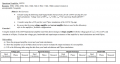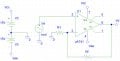# Creating a Voltage Feedback amplifier

#### bokbunja ju

Joined Sep 12, 2015
10
Hey guys, trying to do this for past few days now however not sure whether ive been doing it correct or not. Using microelectronics by sedra smith, ive been trying to work out the values of R1 and Rf. feedback constant = 0.1, due to Beta= R1/ (R1+R2), hence R1 = 9 * R2. Rs and RL was excluded, just like the photo of my circuit for a voltage feedback amplifier. Not sure whether this is right or not :S

*Not sure how to set Pspice up and see if my values have been correct or not.

*#### bokbunja ju

Joined Sep 12, 2015
10
specifically help with Pspice simulations, is there a way to to change internal values to the one suggested in the hints?

#### crutschow

Joined Mar 14, 2008
27,945
You circuit configuration looks correct but I don't understand your gain equations.
What are the "feedback constant", "beta" and R2? Is R2 the same as Rf?

You don't need gain equations. Just note that, due to the op amp's high open loop gain, the voltage between the inputs of an op amp are very near zero when it is operating with negative feedback in the linear region, so use that fact to calculate the values of Rf and R1 you need to get Vo to be 10 times Vs (or Av = 10).

Do you know how to do the calculations for the various required parameters?

In Pspice you do a Transient simulation to simulate the circuit.

•bokbunja ju

#### Jony130

Joined Feb 17, 2009
5,244

#### Attachments

• 3.6 KB Views: 5
Last edited:
•Russmax and bokbunja ju

#### bokbunja ju

Joined Sep 12, 2015
10
@crutshcow, just like jony130 posted. The feedback constant was one of the various parameters needed to find the closed loop gain and etc. Haven't tried this yet Jony130 but will soon as its super late here, thanks. I'll keep you posted about this.

#### bokbunja ju

Joined Sep 12, 2015
10
Acl = Aol/(1 + beta*Aol)

where:

Acl - closed loop gain
Aol - open-loop gain of a op amp ( forward gain )
Beta - feedback factor ( feedback gain ) - V"-"/Vout = R1/(R1 + Rf)

EDIT

For small-signal modeling you can use this VCVS in pspice

View attachment 91454
provided that the open loop gain was 200 V/mV, was this required to be inserted in E1 through model changes?
And in one of my photos, it suggests to find the input/output resistance, bandwidth and voltage gain in Pspice, would this be through graphing or is a results output panel?

#### Jony130

Joined Feb 17, 2009
5,244
provided that the open loop gain was 200 V/mV, was this required to be inserted in E1 through model changes?
Did you open the file ? And notice that I set E gain to 200k
And in one of my photos, it suggests to find the input/output resistance, bandwidth and voltage gain in Pspice, would this be through graphing or is a results output panel?
Using this mode you can find input/output resistance and voltage gain but not the bandwidth.

#### bokbunja ju

Joined Sep 12, 2015
10
Did you open the file ? And notice that I set E gain to 200k

Using this mode you can find input/output resistance and voltage gain but not the bandwidth.
Currently using Pspice 9.1 student, which is required at my university. It seems that it can't be opened or just opens with a blank page.

#### bokbunja ju

Joined Sep 12, 2015
10
i'm currently using a "restricted version, so A size is only permitted"

#### bokbunja ju

Joined Sep 12, 2015
10
Did you open the file ? And notice that I set E gain to 200k

Using this mode you can find input/output resistance and voltage gain but not the bandwidth.
solved the issue with the file, by just recreating it and it seems to be working. the voltage gain required was 10V/V. So i think the resistors should be 1k and 9k instead of 1k and 10k. Can't seem to plot anything to find the resistance. How did you go about this?

#### Jony130

Joined Feb 17, 2009
5,244
Simply try to use a AC sweep analysis, use VSRC source as Vin (set AC = 1) as and plot Rin = Vin/Iin next short the Vin voltage and put a new voltage source (Vx also AC=1) at the output (between Vout and GND) and plot Rout = Vx/Ix

#### JoeJester

Joined Apr 26, 2005
4,390
So i think the resistors should be 1k and 9k instead of 1k and 10k.
Do you know why that is the case?

Besides, you are "restricted" in what resistors you can you.

Last edited:

#### bokbunja ju

Joined Sep 12, 2015
10
thanks everyone, this really helped before we headed in the lab. @joe, there was a needed close loop gain of 10 v/v and when you around resistors close to those ideal values you are able to produce the desired output. Thanks!

#### JoeJester

Joined Apr 26, 2005
4,390
I know there was a closed loop. In one configuration you could have used the 1k and 10k for a 10 v/v, and your choice of using 9 each 10k resistors in series paralleled with a 10 k resistor to form the 9k, in the other configuration. Do you know which configuration requires the 1k and 10k and which requires the 1k and 9k for a 10 V/V?

#### Russmax

Joined Sep 3, 2015
82
You can also use .tf in PSPICE to find the DC small signal values of Rin, Ro, and Av, since you're not using any capacitors to block DC.

Jony130's circuit will give you all your answers except bandwidth. If you have access to the uA741 op amp as a subcircuit model, then use that. If you have a schematic symbol for the uA741, then simply place that, and make sure you are linked to its subcircuit definition in your netlist.

You can also modify Jony130's circuit as follows:
Between E1 and R, insert another VCVS, E2. In- and Out- to GND. Out+ to Rout. Give it a gain of 1.
Between E1 Out+ and E2 In+, insert a resistor, Rbw, value discussed below.
From E2 In+ to GND insert a capacitor, Cbw, value discussed below.

The exact values of Rbw and Cbw don't matter, but you are going to use them to set the Open Loop BW of amplifier.
So Rbw*Cbw = 1/(2*pi*F). This will allow you to simulate to find all required answers. See how they compare to what you measured. Good times.

Regards